G-GMD Categories All categories Not categorized G-C G-CO G-GMD G-GPE G-MD G-SRT Modeling Prioritized

#### MAT-HS.G-GMD.01

 MAT-HS Targeted Standards(G) Concept: Geometry(GMD) Domain: Geometric Measurement and DimensionCluster: Explain volume formulas and use them to solve problems. MAT-HS.G-GMD.01 Give an informal argument for the formulas for the circumference of a circle, area of a circle, volume of a cylinder, pyramid, and cone. Use dissection arguments, Cavalieri’s principle, and informal limit arguments.

## Student Learning Targets:

• I can
• I can

### Reasoning Targets

• I can explain the formulas for circumference of a circle and area of a circle by determining the meaning of each term.
• I can explain the formulas for volume of a cylinder, pyramid and cone by determining the meaning of each term.

• I can
• I can

• I can
• I can

## Proficiency Scale

 Score Description Sample Activity 4.0 In addition to Score 3.0, the student demonstrates in-depth inferences and applications regarding more complex material that go beyond end of instruction expectations. - 3.5 In addition to Score 3.0 performance, the student demonstrates in-depth inferences and applications regarding the more complex content with partial success. 3.0 “The Standard.” The student demonstrates no major errors or omissions regarding any of the information and processes that were end of instruction expectations. - 2.5 The student demonstrates no major errors or omissions regarding the simpler details and processes (Score 2.0 content) and partial knowledge of the more complex ideas and processes (Score 3.0 content). 2.0 The student demonstrates no major errors or omissions regarding the simpler details and processes but exhibits major errors or omissions regarding the more complex ideas and processes (Score 3.0 content). - 1.5 The student demonstrates partial knowledge of the simpler details and processes (Score 2.0 content) but exhibits major errors or omissions regarding the more complex ideas and procedures (Score 3.0 content). 1.0 With help, the student demonstrates a partial understanding of some of the simpler details and processes (Score 2.0 content) and some of the more complex ideas and processes (Score 3.0 content). - 0.5 With help, the student demonstrates a partial understanding of some of the simpler details and processes (Score 2.0 content) but not the more complex ideas and processes (Score 3.0 content). 0.0 Even with help, the student demonstrates no understanding or skill. -

## Resources

 Web Vocab

#### MAT-HS.G-GMD.02

 MAT-HS Targeted Standards(G) Concept: Geometry(GMD) Domain: Geometric Measurement and DimensionCluster: Explain volume formulas and use them to solve problems.  MAT-HS.G-GMD.02 Calculate the surface area for prisms, cylinders, pyramids, cones, and spheres to solve problems.

• I can
• I can

• I can
• I can

• I can
• I can

• I can
• I can

## Proficiency Scale

 Score Description Sample Activity Advanced In addition to Score 3.0, the student demonstrates in-depth inferences and applications regarding more complex material that go beyond end of instruction expectations. Create a solid of revolution by rotating a 2 dimensional figure about a line and find the surface area of that solid. A geometric figure is created when bounded by the lines y=¾ x-3, y=0, x=0.  Draw a picture of the region being revolved about the x-axis.  Determine the surface area of the geometric solid. 3.5 In addition to Score 3.0 performance, the student demonstrates in-depth inferences and applications regarding the more complex content with partial success. Proficient “The Standard.” The student demonstrates no major errors or omissions regarding any of the information and processes that were end of instruction expectations. Calculate the surface area formulas for cylinders, pyramids, cones, and spheres to solve problems Solve for various heights and lengths for use in the surface area formulas using inductive reasoning, pythagorean theorem, and trigonometry. Label the answer using the correct units based on the context of the problem. Give either the approximate or exact answer, given the context of the problem. Find the surface area of the figure: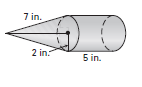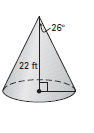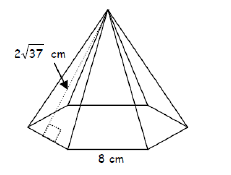2.5 The student demonstrates no major errors or omissions regarding the simpler details and processes (Score 2.0 content) and partial knowledge of the more complex ideas and processes (Score 3.0 content). Progressing The student demonstrates no major errors or omissions regarding the simpler details and processes but exhibits major errors or omissions regarding the more complex ideas and processes (Score 3.0 content). Select the appropriate formula for finding the surface area of a solid Students can solve for surface area of given shapes when given all necessary measurements Find the surface area of the figure: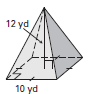1.5 The student demonstrates partial knowledge of the simpler details and processes (Score 2.0 content) but exhibits major errors or omissions regarding the more complex ideas and procedures (Score 3.0 content). Beginning With help, the student demonstrates a partial understanding of some of the simpler details and processes (Score 2.0 content) and some of the more complex ideas and processes (Score 3.0 content). can solve for surface area with help - 0.5 With help, the student demonstrates a partial understanding of some of the simpler details and processes (Score 2.0 content) but not the more complex ideas and processes (Score 3.0 content). 0.0 Even with help, the student demonstrates no understanding or skill. -

## Resources

 Web Vocab

#### MAT-HS.G-GMD.03

 MAT-HS Targeted Standards(G) Concept: Geometry(GMD) Domain: Geometric Measurement and DimensionCluster: Explain volume formulas and use them to solve problems MAT-HS.G-GMD.03 Know and apply volume formulas for prisms, cylinders, pyramids, cones, and spheres to solve problems.

## Student Learning Targets:

### Knowledge Targets

• I can choose the appropriate formula from a list.

### Reasoning Targets

• I can, given formulas, compute the surface area and volume of a right prism, right cylinder, right pyramid, right cone, and sphere.

• I can
• I can

• I can
• I can

## Proficiency Scale

 Score Description Sample Activity Advanced In addition to Score 3.0, the student demonstrates in-depth inferences and applications regarding more complex material that go beyond end of instruction expectations. Create a solid of revolution by rotating a 2 dimensional figure about a line and find the volume of that solid. A geometric figure is created when bounded by the lines y=¾ x-3, y=0, x=0.  Draw a picture of the region being revolved about the x-axis.  Determine the volume of the geometric solid. 3.5 In addition to Score 3.0 performance, the student demonstrates in-depth inferences and applications regarding the more complex content with partial success. Proficient “The Standard.” The student demonstrates no major errors or omissions regarding any of the information and processes that were end of instruction expectations. Know and use volume formulas for cylinders, pyramids, cones, and spheres to solve problems. Solve for various heights and lengths for use in the volume formulas using inductive reasoning, pythagorean theorem, and trigonometry. Label the answer using the correct units based on the context of the problem. Give either the approximate or exact answer, given the context of the problem. Find the volume of the figure:Find the value of x.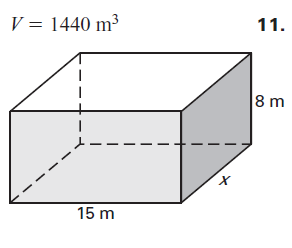2.5 The student demonstrates no major errors or omissions regarding the simpler details and processes (Score 2.0 content) and partial knowledge of the more complex ideas and processes (Score 3.0 content). Progressing The student demonstrates no major errors or omissions regarding the simpler details and processes but exhibits major errors or omissions regarding the more complex ideas and processes (Score 3.0 content). Select the appropriate formula for finding the volume of a solid. Students can solve for volume of given shapes when given all necessary measurements Find the volume of the figure:1.5 The student demonstrates partial knowledge of the simpler details and processes (Score 2.0 content) but exhibits major errors or omissions regarding the more complex ideas and procedures (Score 3.0 content). Beginning With help, the student demonstrates a partial understanding of some of the simpler details and processes (Score 2.0 content) and some of the more complex ideas and processes (Score 3.0 content). Students can solve for volume with help. 0.5 With help, the student demonstrates a partial understanding of some of the simpler details and processes (Score 2.0 content) but not the more complex ideas and processes (Score 3.0 content). 0.0 Even with help, the student demonstrates no understanding or skill. -

## Resources

 Web Vocab

#### MAT-HS.G-GMD.04

 MAT-HS Targeted Standards(G) Concept: Geometry(GMD) Domain: Geometric Measurement and DimensionCluster: Visualize relationships between two-dimensional and three-dimensional objects MAT-HS.G-GMD.04 Identify the shapes of two-dimensional cross-sections of three-dimensional objects, and identify three-dimensional objects generated by rotations of two-dimensional objects.

## Student Learning Targets:

### Knowledge Targets

• I can identify the properties and attributes of two and three-dimensional objects that distinguish one from another; e.g., a square is a parallelogram with four congruent sides and four right angles; a cylinder has two parallel circular bases.
• I can identify two dimensional cross sections of three dimensional objects.
• I can identify three dimensional objects created by rotating two dimensional objects.

• I can
• I can

• I can
• I can

• I can
• I can

## Proficiency Scale

 Score Description Sample Activity 4.0 In addition to Score 3.0, the student demonstrates in-depth inferences and applications regarding more complex material that go beyond end of instruction expectations. - 3.5 In addition to Score 3.0 performance, the student demonstrates in-depth inferences and applications regarding the more complex content with partial success. 3.0 “The Standard.” The student demonstrates no major errors or omissions regarding any of the information and processes that were end of instruction expectations. - 2.5 The student demonstrates no major errors or omissions regarding the simpler details and processes (Score 2.0 content) and partial knowledge of the more complex ideas and processes (Score 3.0 content). 2.0 The student demonstrates no major errors or omissions regarding the simpler details and processes but exhibits major errors or omissions regarding the more complex ideas and processes (Score 3.0 content). - 1.5 The student demonstrates partial knowledge of the simpler details and processes (Score 2.0 content) but exhibits major errors or omissions regarding the more complex ideas and procedures (Score 3.0 content). 1.0 With help, the student demonstrates a partial understanding of some of the simpler details and processes (Score 2.0 content) and some of the more complex ideas and processes (Score 3.0 content). - 0.5 With help, the student demonstrates a partial understanding of some of the simpler details and processes (Score 2.0 content) but not the more complex ideas and processes (Score 3.0 content). 0.0 Even with help, the student demonstrates no understanding or skill. -

## Resources

 Web Vocab